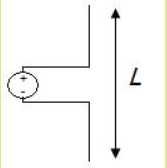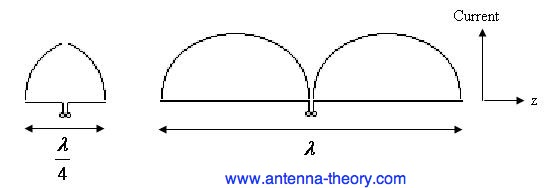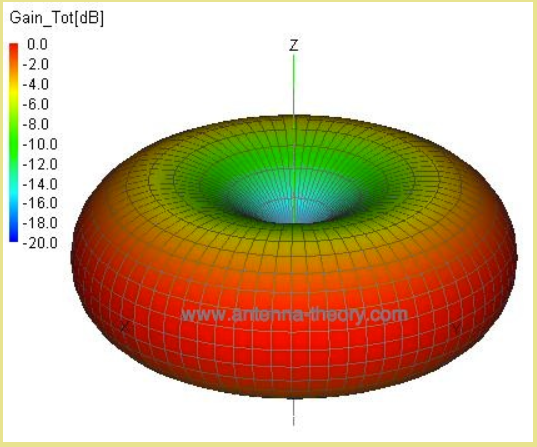### Type of Antennas

1. Wire Antennas

2. Micro strip Antennas

3. Aperture Antennas

4. Reflector Antennas

5. Log periodic Antennas

6. Lens Antennas

7. Travelling wave antennas

8. Micro strip Antennas

9. Aperture Antennas

10. Reflector Antennas

11. Log periodic Antennas

12. Lens Antennas

TYPE OF ANTENNAS

• Antennas must be categorised in order to fully comprehend their physical structure and functionality. Antennas come in a variety of shapes and sizes, depending on the use.These are basically divided into seven categories with examples below;

• Wire Antennas

• Power passes from one end of the wire to the other end of the transmission line. If both ends of a transmission line are connected to circuits, data will be transmitted or received between these two circuits utilising this wire.

• The power in this cable tries to escape if one end of it is not connected. As a result, wireless communication is possible. When one end of the wire is bent, more energy tries to escape from the transmission line than before. Radiation is the term used for this deliberate escape.

• Some examples of wire antenna are below;

1. Short dipole
• The simplest of all antennas is the short dipole. It's only an open-circuited wire with a feed in the middle, as shown in Figure below..

• In antenna engineering, the adjectives "short" or "tiny" always mean "relative to a wavelength." As a result, the size of the wire relative to the wavelength of the operating frequency is more important than the size of the dipole antenna itself. A dipole is considered short if it is less than a tenth of a wavelength long.Figure: short dipole

• This current oscillates at frequency f in time sinusoidally. Figure below shows the current distributions for quarter-wavelength (left) and full-wavelength (right) dipole antennas. It's worth remembering that the current doesn't reach its maximum value until the dipole is longer than half a wavelength.• wavelength (right) dipole antennas. It's worth remembering that the current doesn't reach its maximum value until the dipole is longer than half a wavelength.

​​​​​​​​​​​​​​Figure: Current distributions on finite-length dipole antennas.

• It's worth noting that the input impedance of very small dipole antennas is capacitive, which means the impedance is dominated by a negative reactance value (and a relatively small real impedance or resistance). The input resistance rises with the size of the dipole, as does the reactance. The antenna is considered to be resonant when the imaginary component of the impedance of the antenna is less than 0.5 lambda (reactance X=0).

• When the length of the dipole antenna approaches one wavelength, the input impedance becomes infinite. High frequency transmission line theory helps explain this erratic shift in input impedance. Consider the one-wavelength dipole given in the above Figure as an example.

​​​​​​​​​​​​​​Figure: Input impedance as a function of the length (L) of a dipole antenna

• The quarter-wavelength dipole antenna is less directional than the full-wavelength dipole antenna. This is a common finding in antenna theory: increasing directivity requires a larger antenna in general. The outcomes, on the other hand, are not always evident. Figure also shows the 1.5-wavelength dipole pattern. It's worth noting that this pattern peaks at +45 and -45 degrees.

• When viewed azimuthally (around the dipole's long axis), the dipole antenna is symmetric, hence the radiation pattern is not a function of the azimuthal angle azimuth angle phi. As a result, the dipole antenna is an omnidirectional antenna. Furthermore, because the E-field has only one vector component, the fields are linearly polarised.​​​​​​​

Figure: 3D pattern of one wavelength dipole antenna

• Whereas the 3d radiation pattern of 1.5 wavelength dipole antenna is totally different as shown in figure below.​​​​​​​

Figure: 3D normalised radiation pattern of a 1.5 wavelegth dipole

1. half-wave dipole antenna

The half-wave dipole antenna is merely a variant of the dipole antenna, but it is significant enough to warrant its own section. The term "half-wave" refers to the length of this dipole antenna being equal to a half-wavelength at the operating frequency.
To be clear, what size half-wavelength dipole should the antenna have if it is to broadcast at 600 MHz?
Lambda = 𝝀 = c / f = 0.5 metres is one wavelength at 600 MHz. As a result, the length of a half-wavelength dipole antenna is 0.25 metres (or 9.85 inches if you prefer not to use the metric system).

As you might assume, the half-wave dipole antenna is a simple half-wavelength wire fed at the centre, as shown in Figure below.​​​​​​​